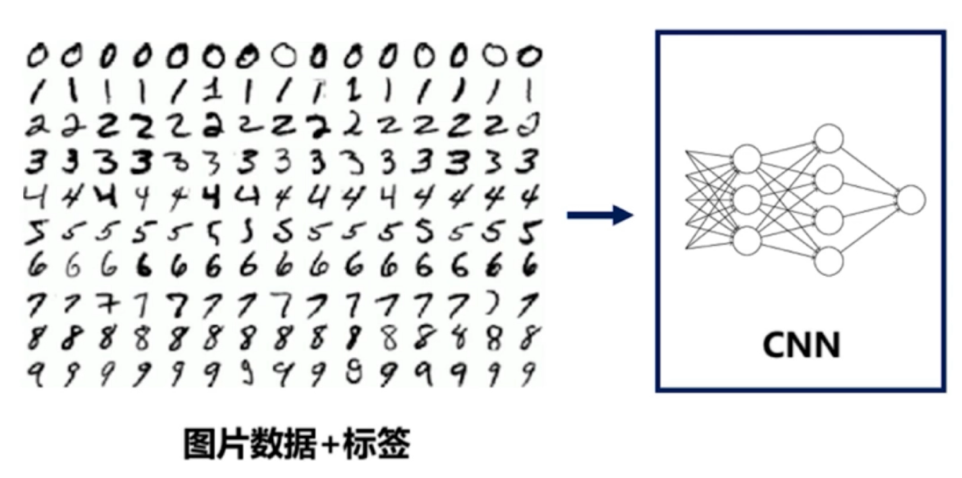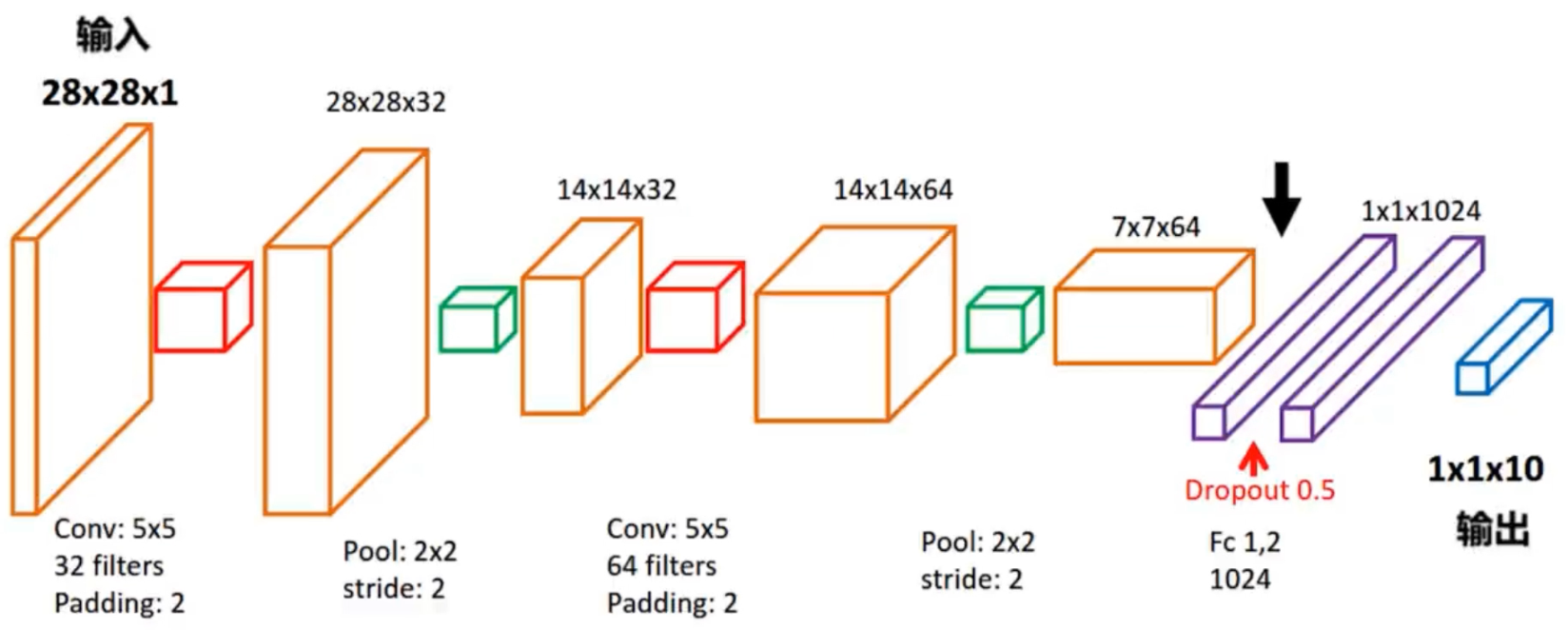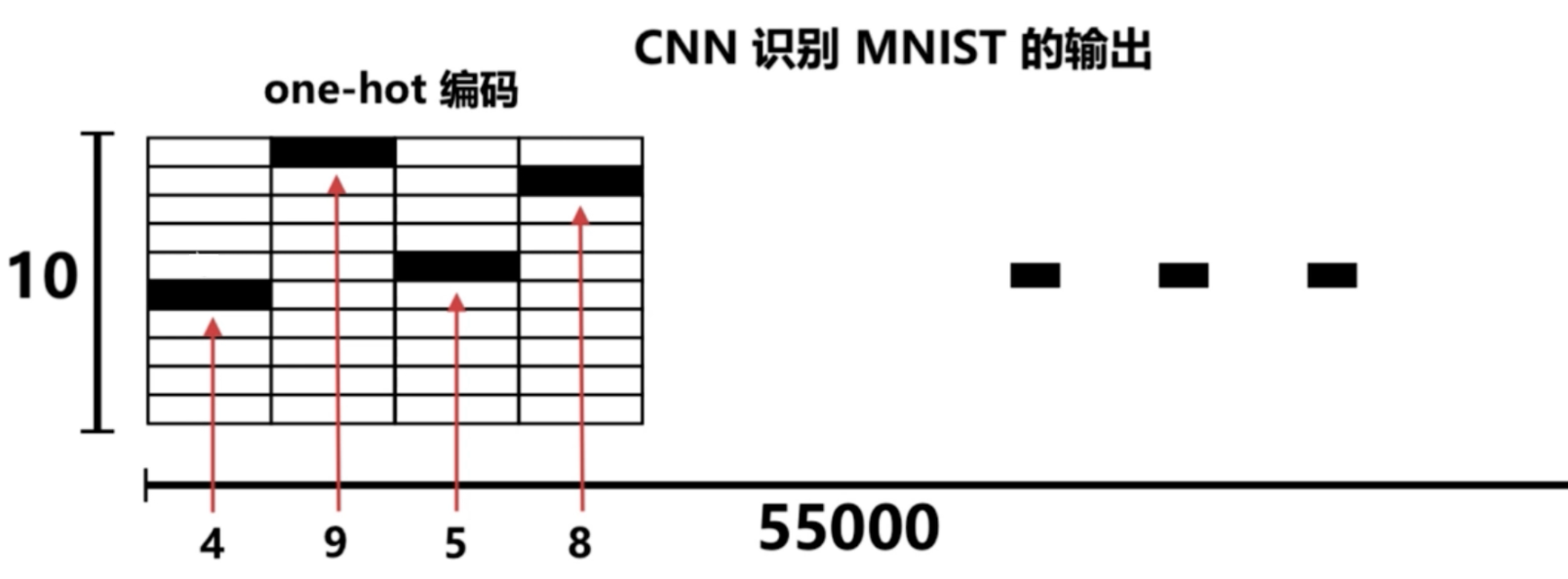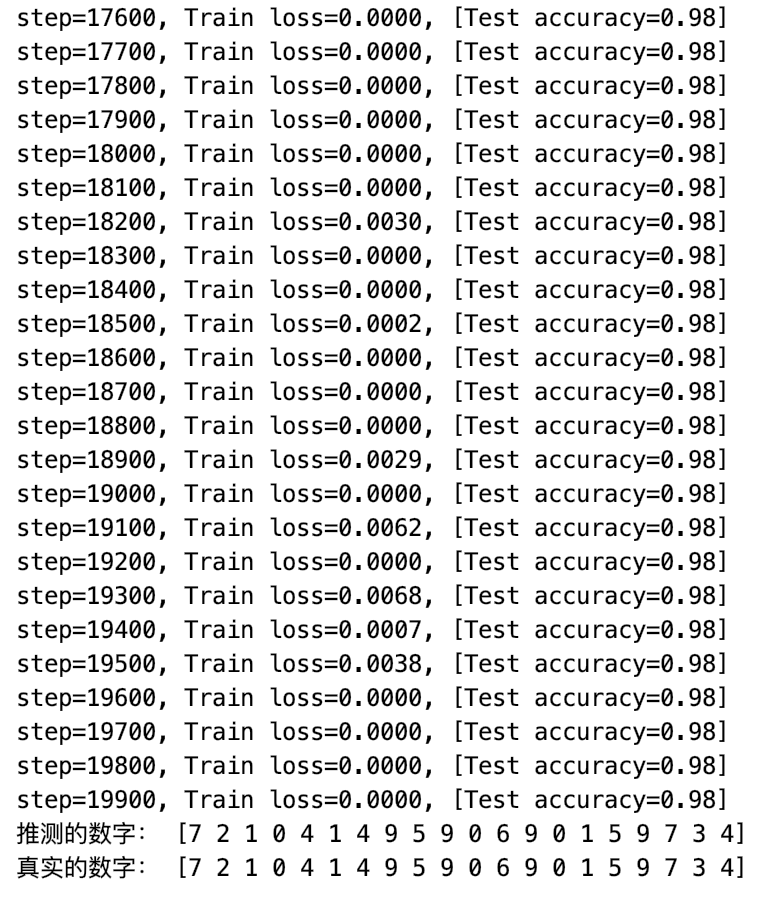# Python遇见机器学习 ---- TensorFlow搭建神经网络实现手写识别0 -> 1000000000

1 -> 0100000000

2 -> 0010000000

3 -> 0001000000

4 -> 0000100000

5 -> 0000010000

6 -> 0000001000

7 -> 0000000100

8 -> 0000000010

9 -> 0000000001### 卷积层tf.layers.conv2d：

``````tf.layers.conv2d(
inputs,
filters,
kernel_size,
strides=(1, 1),
activation=None,
)``````
• inputs: 张量输入
• filters: 整数，输出空间的维数(即卷积中滤波器的个数)
• kernel_size: 一个整数或两个整数的元组/列表，指定二维卷积窗口的高度和宽度。可以是单个整数，以便为所有空间维度指定相同的值
• strides: 一个整数或两个整数的元组/列表，指定卷积在高度和宽度上的步长。可以是单个整数，以便为所有空间维度指定相同的值。指定任何stride值!= 1与指定任何dilation_rate值!= 1不兼容
• padding: "valid" 或 "same" (不区分大小写)
• activation: 激活函数。将其设置为None为保持线性激活

``````# 第1层卷积
conv1 = tf.layers.conv2d(
inputs=input_x_images,  # 形状28*28*1
filters=32,             # 输出深度为32
kernel_size=[5, 5],     # 滤波器在二维的大小
strides=1,              # 采样步长
activation=tf.nn.relu
)       # 输出形状变为[28, 28, 32]``````

### 池化层（亚采样）tf.layers.max_pooling2d：

``````tf.layers.max_pooling2d(
inputs,
pool_size,
strides,
)``````
• inputs: 要池化的张量。必须是4阶
• pool_size: 指定池窗口大小的整数或两个整数的元组/列表:(pool_height, pool_width)。可以是单个整数，以便为所有空间维度指定相同的值
• strides: 一个整数或两个整数的元组/列表，指定池操作的步长。可以是单个整数，以便为所有空间维度指定相同的值

``````# 第1层池化（亚采样）
pool1 = tf.layers.max_pooling2d(
inputs=conv1,           # [28,28,32]
pool_size=[2, 2],
strides=2,
)       # 形状[14, 14, 32]``````

``````# 第2层卷积（亚采样）
conv2 = tf.layers.conv2d(
inputs=pool1,           # 形状[14,14,32]
filters=64,             # 输出深度为64
kernel_size=[5, 5],     # 滤波器在二维的大小
strides=1,              # 采样步长
activation=tf.nn.relu
)       # 形状[14, 14, 64]``````

``````# 第2层池化（亚采样）
pool2 = tf.layers.max_pooling2d(
inputs=conv2,           # [14,14,64]
pool_size=[2, 2],
strides=2,
)       # 形状[7, 7, 64]``````

``flat = tf.reshape(pool2, [-1, 7 * 7 * 64])      # 形状[7*7*64, ]``

### 密集连接层 tf.layers.dense：

``````tf.layers.dense(
inputs,
units,
activation=None,
)``````
• inputs: 张量输入
• units: Integer型或 Long型, 输出空间的维度
• activation: 激活函数(调用)。将其设置为None为线性激活

1024个神经元的全连接层定义如下：

``dense = tf.layers.dense(inputs=flat, units=1024, activation=tf.nn.relu)``

``dropout = tf.layers.dropout(inputs=dense, rate=0.5)``

10个神经元的全连接层，使用线性激活函数：

``logits = tf.layers.dense(inputs=dropout, units=10)       # 输出 [1,1,10]``

``````# 计算误差（计算Cross entropy（交叉熵），再用softmax计算百分比概率）
loss = tf.losses.softmax_cross_entropy(onehot_labels=output_y, logits=logits)-- 展开阅读全文 --

« 上一篇 07-24